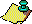Contents Next: Remark. Up: Classical Results Interpreted Previous: Aperiodic Points

We showed earlier that the separation of orbits initially close to each other occurred at an exponential rate. We would like to examine the Lyapunov exponents of the Gauss map, to see if we can explicitly measure the rate of separation. The Lyapunov exponents of orbits of the Gauss map are defined as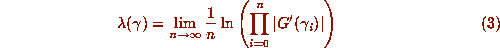whenever this limit exists. Note that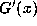exists even at the jump discontinuities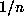, but there is a real singularity at the origin. Nearby orbits will separate from the orbit of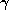at an average rate of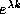, after k iterations of G.

Khintchin  derived a remarkable theorem with which we could show the common Lyapunov exponent of almost all (in the sense of Lebesgue measure) orbits is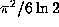. Easier ways have since been found to establish this result, using ergodic theory. We summarize the ergodic results in the next section. Note that for any rational initial point, the above limit does not exist (because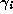is eventually 0 and the derivative blows up there). Further, for any periodic orbit the calculation can be made explicitly, to give Lyapunov exponents that differ from the almost-everywhere value.

For example, the fixed points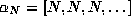have Lyapunov exponents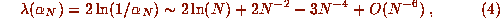so there are orbits with arbitrarily large Lyapunov exponents, i.e., orbits that are arbitrarily sensitive to perturbations in the initial point. The asymptotic formula above was derived from the explicit form for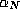obtained by solving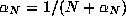for its positive root, and then using Maple's `asympt` command. It is not too hard to show, because the limit can be written down explicitly, that for the orbit of e, the limit defining the Lyapunov exponent is infinite. On the other end of the scale, the special case N=1 ofgives, the golden ratio. Thus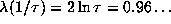, which is smaller than the almost-everywhere Lyapunov exponent. In fact, we have the following:

### Theorem 3

No orbit of the Gauss map has a Lyapunov exponent smaller than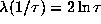.

### [Proof]Contents Next: Remark. Up: Classical Results Interpreted Previous: Aperiodic Points